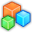# Microsoft Small BasicProgram Listing: NMF299
eqstart:
RAW=0
total=100
percentage=0
TextWindow.WriteLine("What is the name of the subject?, "+name)
TextWindow.WriteLine("What is your score in "+subject+"?, " +name)
TextWindow.WriteLine("what is your highest possible score in the "+subject+" activity " +name+"?")
percentage = (RAW/total)*50+50
TextWindow.WriteLine(percentage)

IF (percentage < 75) Then
ElseIf (percentage >= 75 and percentage < 80) Then
ElseIf (percentage >= 80 and percentage < 85) then
ElseIf (percentage >= 85 and percentage < 90) then
Elseif (percentage >= 90 and percentage < 95) then
Elseif (percentage >= 95 and percentage <= 100) then
EndIf

TextWindow.WriteLine("Well "+name+ " This is your percent "+percentage+ " and the Letter equivalent is " +Grade)

TextWindow.WriteLine("Do you want to compute for another grade "+name+"?")
TextWindow.WriteLine("Type Y for yes or N for no")